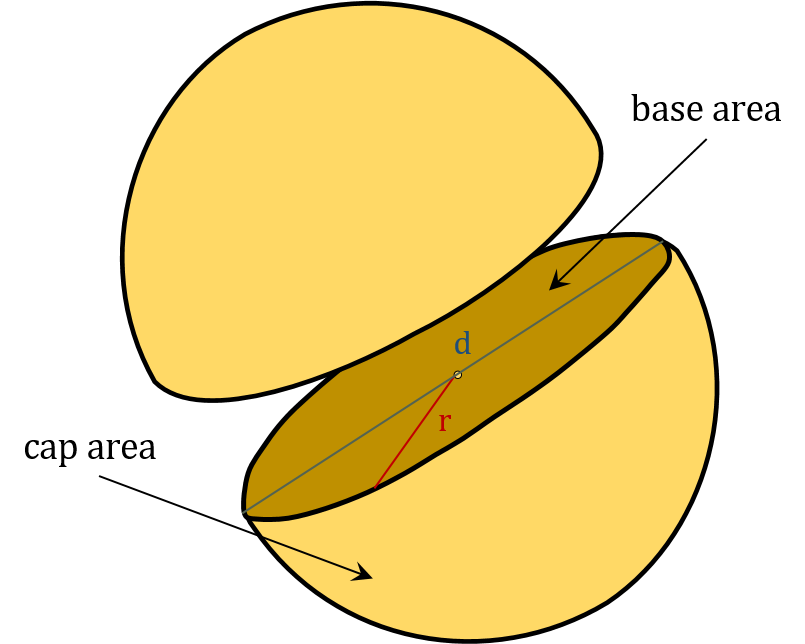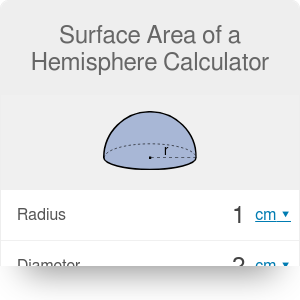in
Diameter
in
Volume
cu in
Surface to volume ratio
1/
in
Surface areas
Base
in²
Cap
in²
Total
in²

# Surface Area of a Hemisphere Calculator

By Dominik Czernia, PhD candidate

Our surface area of a hemisphere calculator is a handy tool that finds different types of hemisphere surface areas. Are you looking for an answer to the question of how to find the surface area of a hemisphere? Or maybe you just need to estimate it quickly? Whatever you plan to do, try this hemisphere calculator which comprises several various area of a hemisphere formulas.

Hemispheres are created by dividing a sphere into two equal halves, as you can see in the picture below. Unlike the full sphere, a hemisphere has two kind of surface areas: the base area (which is a circle) and the cap area. The notation, which we have used in this surface area of a hemisphere calculator, is as follows:• r - radius of a hemisphere,
• d - diameter of a hemisphere,
• V - volume of a hemisphere,
• A - total surface area of a hemisphere,
• Ab - base surface area of a hemisphere,
• Ac - cap surface area of a hemisphere,
• A / V - surface to volume ratio of a hemisphere.

The interesting fact is that the total volume of two hemispheres equals the volume of a one full sphere. However, the same is not true with surface areas. The total area of two hemispheres is greater than the area of a sphere. The reason is simple: hemispheres have an additional base area.

## How to find the surface area of a hemisphere?

When we split a sphere in half and take one of the resulting parts, we get a hemisphere. In each hemisphere, we can name two surface areas: base area and cap area (look at the picture above). From the area of a sphere calculator we know that the surface area of a sphere is as below:

`A(sphere) = 4 * π * r²`.

You can think about it like two times the cap surface area of a hemisphere. Therefore, the hemisphere cap area equals:

`Ac = A(sphere) / 2`,

`Ac = 2 * π * r²`.

The base surface area is a circle with the same radius as a hemisphere. Thus, it can be expressed as:

`Ab = π * r²`.

Finally, the total surface area is the sum of those two contributions:

`A = Ac + Ab`,

`A = 2 * π * r² + π * r²`,

`A = 3 * π * r²`.

This area of a hemisphere calculator allows you to find all three types of surface areas of a specific hemisphere. Moreover, you can do every calculation in different units (SI and imperial). If you want to learn more about area unit conversion, check out our area converter!

## What's the area of a hemisphere formula?

Now, after we know what are the surface areas of a hemisphere and how to find them, let's try to derive different area of a hemisphere formulas. They can be useful in situations when we don't have the radius given. First of all, there are some basic hemisphere equations you should know:

1. Diameter of a hemisphere: `d = 2 * r`,
2. Volume of a hemisphere: `V = 2/3 * π * r³`,
3. Base surface area of a hemisphere: `Ab = π * r²`,
4. Cap surface area of a hemisphere: `Ac = 2 * π * r²`,
5. Total surface area of a hemisphere: `A = 3 * π * r²`,
6. Surface to volume ratio of a hemisphere: `A / V = 9 / (2 * r)`.

The area of a hemisphere formula (for the total area) can be then derived from the above equations. We can obtain even six of them! The equations used by this area of a hemisphere calculator are as follows:

1. Given radius: `A = 3 * π * r²`,
2. Given diameter: `A = 3/4 * π * d²`,
3. Given volume: `A = ³√[243/4 * π * V²]`
4. Given base area: `A = 3 * Ab`,
5. Given cap area: `A = 3/2 * Ac`,
6. Given surface to volume ratio: `A = 243 * π / (4 * (A/V)²)`.

As you know, the Earth is approximately a sphere with a radius of almost 6400 km, in which we can specify the northern and the southern hemispheres (specifically speaking, the Earth is a geoid). This division plays an essential role in geography and physics. For example, there is a force called Coriolis effect which acts on you whenever you travel by airplane, causing you to get off the course. When you're on the northern hemisphere, you will deflect to the right, and on the southern hemisphere to the left.

Dominik Czernia, PhD candidate

## Get the widget!

Surface Area of a Hemisphere Calculator can be embedded on your website to enrich the content you wrote and make it easier for your visitors to understand your message.

It is free, awesome and will keep people coming back!